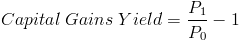# Capital Gains YieldThe formula for the capital gains yield is used to calculate the return on a stock based solely on the appreciation of the stock. The formula for capital gains yield does not include dividends paid on the stock, which can be found using the dividend yield. The capital gains yield and dividend yield is combined to calculate the total stock return.

The capital gains yield formula uses the rate of change formula. Calculating the capital gains yield is effectively calculating the rate of change of the stock price. The rate of change can be found by subtracting an ending amount from the original amount then divided by the original amount.

The capital gains yield will equal a company's total stock return if a company does not pay dividends. A company that pays no dividends will have a 0% dividend payout ratio, a 100% retention ratio, and a 0% dividend yield.

## Capital Gains Yield for Multiple Periods

It is important to remember that if the yields for multiple periods are known, they can not be simply summed to determine the yield for the entire period. For example, if the monthly yields are known, one can not simply add the yields to determine the annual capital gains yield. The holding period return formula must be used when calculating a yield over multiple periods.

## Alternative Capital Gains Yield FormulaThe capital gains yield formula can also be stated as the ending price divided by the initial price then minus one. This alternative formula is a rearranging of the capital gains yield formula shown at the top of the page.

The capital gains yield formula can also be stated aswhich is another way of stating a change in (Delta) price divided by the original stock price.

New to Finance?